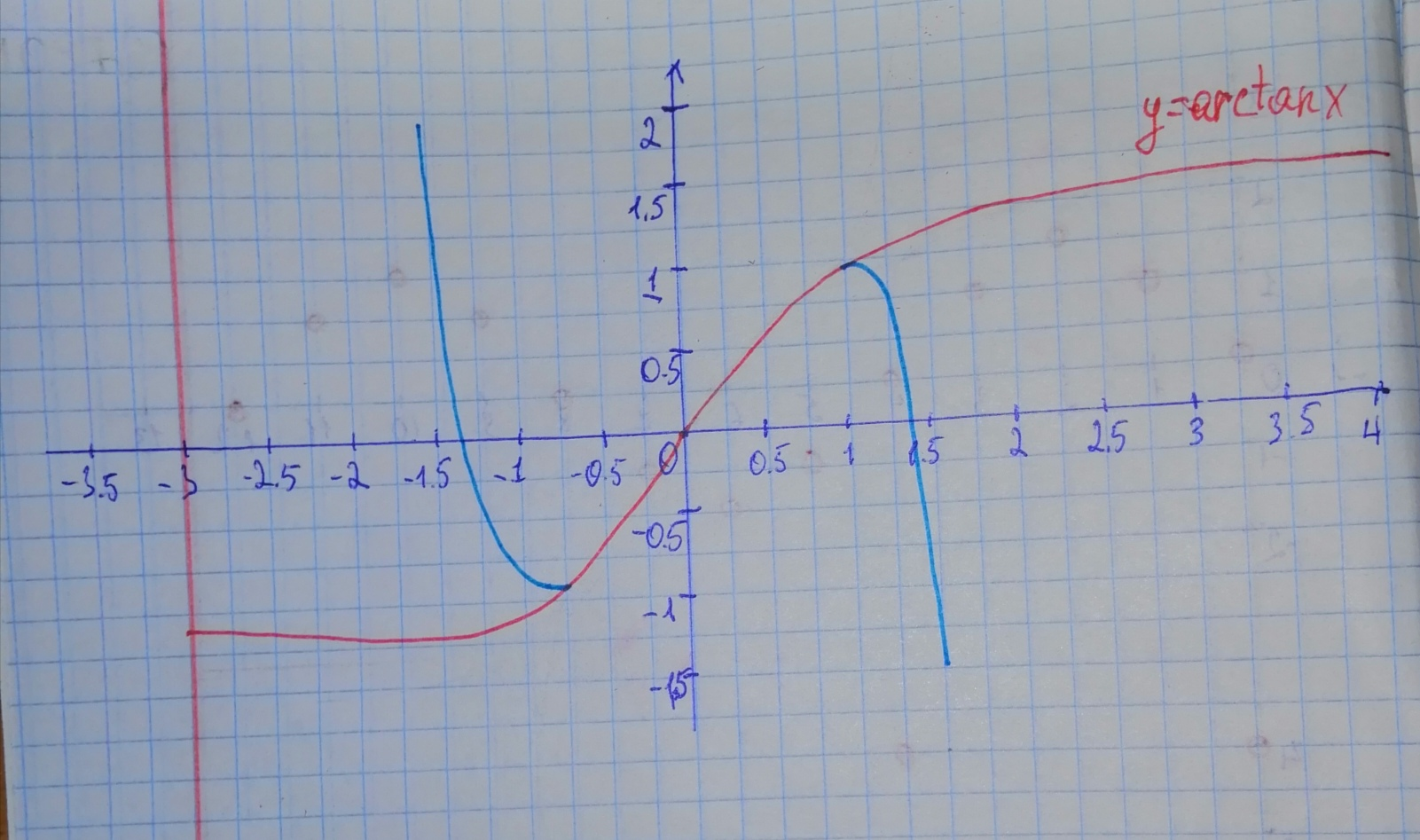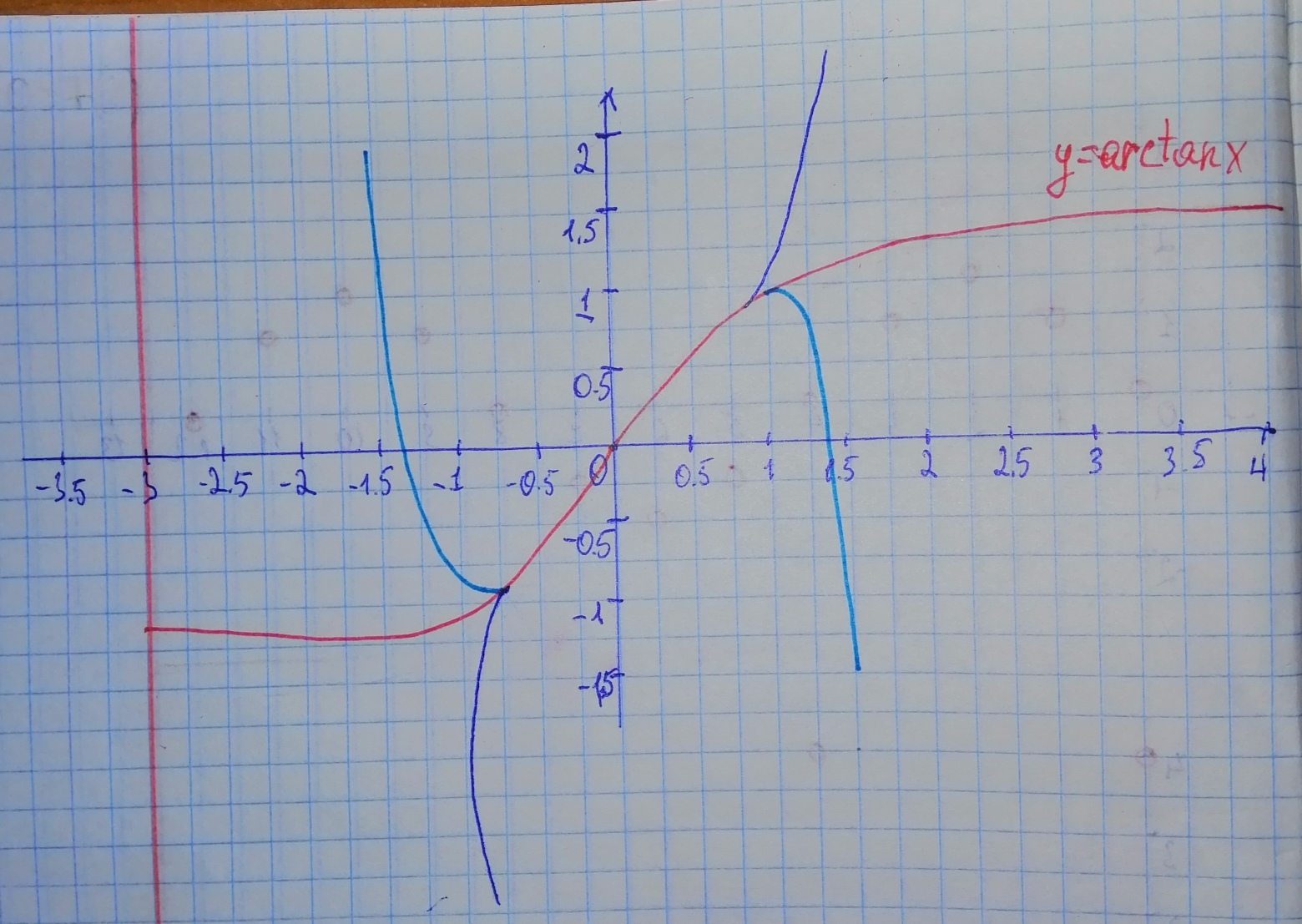# Using calculus, it can be shown that the arctangent function can be approximated by the polynomial arctan x approx x - frac{x^{3}}{3} + frac{x^{5}}{5}tinfoQ 2020-11-05 Answered
Using calculus, it can be shown that the arctangent function can be approximated by the polynomial

a) Use a graphing utility to graph the arctangent function and its polynomial approximation in the same viewing window. How do the graphs compare?
b) Study the pattern in the polynomial approximation of the arctangent function and predict the next term. Then repeat part (a). How does the accuracy of the approximation change when an additional term is added?
You can still ask an expert for help

• Questions are typically answered in as fast as 30 minutes

Solve your problem for the price of one coffee

• Math expert for every subject
• Pay only if we can solve itbahaistag

Step 1
a)Step 2
a) (blue graph)
Between from about to 1, the polynomial approximation is close to the graph of
Step 3Step 4
b) Based on the pattern, the next exponent is 9, denominator is 9, and the term is positive.
(magenta graph)
Now the approximation stays closer to over a sligghtly wider interval.